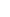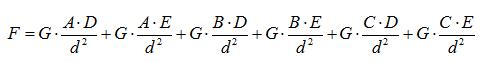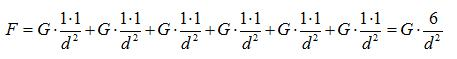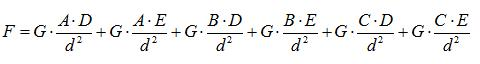29. RELATIONSHIP OF (C+V) (C-V) MATHEMATICS WITH GRAVITATIONAL FORCE AND CHARGE FORCES(c+v) (c-v) mathematics gives important clues on the existence of a mechanism in nature. This mechanism is called FIELD mechanism. Lets have a look at these two equations.Gosh where do these come from? What is it got to do with it? One is the Law of Universal Gravitation and the other is Coulombs Law that describes the force between static electrical charges. What do we have these here when our topic is (c+v) (c-v) mathematics? Please dont say these because these two equations have a close relationship with (c+v) (c-v) mathematics.The Law of Universal Gravitation: Two objects apply forces directly proportional with the multiplication of their masses and inversely proportional to the square of the distance between them.Coulombs Law: Two objects that have electrical charges apply forces directly proportional with the multiplication of their charges and inversely proportional to the square of the distance between them.Both laws include some vital information that guide us and that we may use to explain the reason of (c+v) (c-v) mathematics. Lets have a look at that information now.The first piece of information is the following: Valid for both the equations, there is no limit to the distance between two objects or two electrical charges. The distance difference, whether it is one centimeter, one meter, thousands of kilometers, a few light years or millions of light years, does not lead to a change in these equations.The second piece of information is that features of objects are not important. There is no limitation such as only and only objects included in this class apply forces to each other. It is enough for the Law of Universal Gravitation to have objects that have masses and for Coulombs Law to have objects that have electrical charges.The third piece of information is that these forces are applied to all objects that are in the universe simultaneously instead of specific objects. I mean, there is no limit such as while an object is applying forces to another object, it cannot apply forces to another object. While Earth applies forces to the Moon, it also applies forces to the Sun, Jupiter, Pluto, and the Andromeda Galaxy or to a celestial body that we can see with Hubble Telescope millions of light years away. It was a bit general example, so be more specific. The Law of Universal Gravitation says that an atom, since it carries mass, apply forces to all the other atoms no matter where they are in the universe. Coulombs Law says that an electron, since it carries a charge, apply forces to all the other electrons and protons no matter where they are in the universe. Here, the fact that an electron that applies forces is inside a neutral atom has no importance (Neutral Atom: The negatively charged electrons and the positively charged protons that form an atom are matched with one another and, for this reason, the electrical charge of an atom is theoretically zero.). It is, in fact, a situation that is so interesting and that forces our imagination as much as possible. You switch on the light in your room at home, electrons start running in a wire towards the lamp and all the other electrons on the other side of the universe are informed about this. How many electrons are running and what their speeds are they know it all (or they will, lets say)! Id like to tell you a weirder thing. And there is also a possibility that the delivery of relocation information to other objects takes place in zero time. I didnt say this to start an argument; Im just trying to tell what the equations mean. If we are careful, we can see that both equations are independent of the speeds of objects. At the moment an object changes its place, the distances it has between the other objects will change and, for this reason, the force will change, as well. We can interpret this situation as The change in the force takes place in zero time. Therefore, if these equations always have absolute accuracy, there is also a result meaning that relocation information of an object is delivered to all other objects in zero time. If it is not delivered in zero time, it means that there is a flaw, a neglected part, in the writing of the equations. And, in this case, the equations that indicates the force between two objects that are motionless relative to each other accurately but that fall short for the forces between objects that are in motion. This is not something that may not happen. If you remember a very special example similar to this, we saw it in the equation c = f0.λ0 that was used for the electromagnetic wave speed equation and the correct equation had to be c = f0.λ1.The fourth information is that these forces stop at nothing and can go through matter. Lets me explain it with a simple example. When Earth goes between the moon and the sun, does the force that the sun applies to the moon change? Does Earth prevent the force of the Sun to reach the Moon? If something that happened, the orbit of the Moon would change and we would detect and notice it. The situation is like this for the gravitational force at least; I think it might be a bit different for charge forces because we can get isolated areas that electrical charges cannot go through.Now Id like to show you that gravitational force stops at nothing. For this, Id like to explain what "m1.m2" multiplication in the equation of the Law of Universal Gravitationmeans with an example.We have 5 shots (metal balls) each weighing one kilogram. By attaching three of them together we get mass m1 and the other two together we get mass m2. The gravitational force between these two masses is F=G. (3x2)/d2. And m1.m2 = 3x2 = 6. Firstly, what is the meaning of the number 6 that we got by multiplying the masses? Im going to try to explain this. Then we will focus on the distance d between two masses.We multiplied the amounts of masses to find the gravitational force , but now lets do it in a more sensitive way: calculate gravitational force of each shot that forms the m1 mass with each shot in m2 mass  and add up the results that we get and find the gravitational force in this way .m1 is made up of (A,B,C) elements and m2 is made up of (D,E) elements. Using this information and the average distance for the distance, an equation like the following is obtained.As seen below, we get the same result with the force that we calculated by using the elements for the m1.m2 multiplication of masses. The masses of the elements in this example were 1 unit.In reality, how many elements the masses m1 and m2 are made up of or what the mass values of the elements are do not matter for the result. I prepared the table below to show this. In the table below, it is assumed that m1 mass is 19 units and m2 mass is 9 units. The elements that make up of m1 and m2 are given random mass values. m1.m2 multiplication gives us 19x9=171. As seen in the table, the addition of the multiplication of the elements with each other is also equal to 171 (the sum of the numbers in the yellow section).As can be understood from the table, the important thing for the gravitational force and the Electrical Charge Force is the sum of the forces that elements of an object apply to the elements of the other object. The resulting force is made up of this sum. The functioning mechanism of nature is this way. Matter is made up of atoms. Therefore, elements that constitute mass are atoms (We can go into a deeper level; we can also say that matter is made up of sub-atomic particles that constitute atoms, but lets stick to the atomic scale.). If we assume that Earth is made up of around 1.33*1050 atoms and the Moon is made up of roughly 1.33*1048 atoms and if we think that each atom on Earth applies gravitational force to each atom on the Moon, we can see how crazy things nature does. Besides, it is estimated that the universe is made of 1081 atoms. From this, we can conclude that each and every atom applies forces to 1081 atoms at the same time.Now, we should focus on the topic of the distance between. While showing the force between elements, I used the average distance above . It is clear that the force calculated by using the distances between elements will give the real result .In the gravitational force equation, the distances between the objects centers of masses are the reference points .In the calculation done with the elements, on the other hand, the accuracy of the equation will increase as the distances will be taken into consideration in a very sensitive manner. But, when the distances are taken into account for both calculations, is there a difference between the results obtained? If yes, how much is this difference?I wrote a special computer program to find the answer to this question. In the program, I did the necessary calculations by using 9000 points as elements for each mass. The mass of an element was taken as 1 unit. You can see the result in the table below.FN : Universal Gravitational Force. The force that is calculated with the regular formula FE : The force that is calculated with the help of distances between elements of two massesAs can be seen in the table, long distances for FN and FE forces give the same results in both calculations. In reality, there can never be FN = FE. However, as we can see, as the distances get longer, the difference between forces decreases and it turns into an unimportant situation like at how many digits after the comma the equation becomes insignificant.As the distances between masses get shorter, the opposite is the case. We see that the difference between FN and FE forces increase and that FE force gradually get higher values. Naturally, the existence of this situation should change our perspective of gravitational force. For instance, while studying movements of planets in orbits that are close to stars, it is important to think how accurate is to calculate by using FN force. FE is at work during stellar collisions, as well. In these types of analyses, it is necessary to take FE force into consideration instead of FN force. FE is the force that affects us while we go and measure our weights on a scale.The model I did is 2-dimensional: 900 points that I used for the masses and 90002 = 81.000.000 number of processes is enough to give us an average idea. I believe it is a real necessity to reach a lot more sensitive information with more points and with 3-dimensional models.What I told you above are the conclusions that I reached from the equation.Of course, the universal gravitational force equation includes other information as well. For example, Why is the force inversely proportional to the square of distance? I cannot answer this question. What is the Universal Gravitational Constant indicated by G? I cannot answer this one, either. I had worked on the G constant for a while before. I cant say I reached any significant information. However, if we think that the descriptions of second, mass and meter are created artificially, we can reach some conclusions.There are three important details in what we covered here in terms of our topic.   Effects of these forces reach infinite distances.  Total force is formed from elements.   These forces stop at nothing and they can pass through matter.  I wanted to show you these. Now, lets go back to our topic.